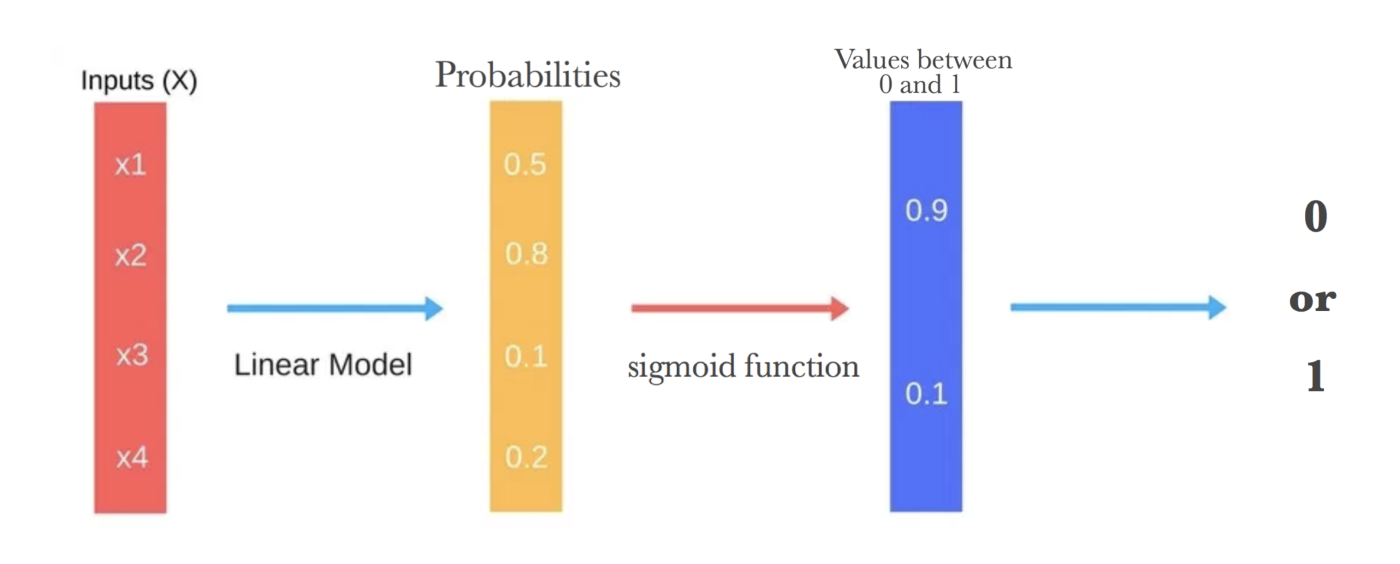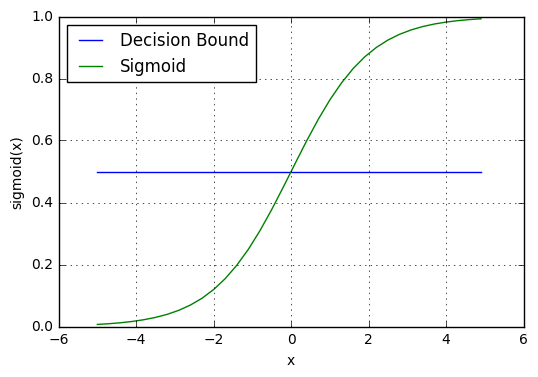# Logistic Regression

## What is Logistic Regression?

Logistic Regression is a statistical model used to determine if an independent variable has an effect on a binary dependent variable. This means that there are only two potential outcomes given an input. For example, it may be used to determine if an email is spam, or not, using the rate of misspelled words, a common sign of spam. Other forms of regression analysis, like a linear regression, require the definition of a threshold to distinguish the binary classes (e.g. <50% misspelled = not spam, >50% misspelled= spam). Linear regression allows for a probability to be established, but it must then be applied to a logistic regression to make the distinct classification.Source

## How does Logistic Regression work?

A commonly used model is a sigmoid function. In the sigmoid function, also known as a squashing function, outputs are contained between the boundaries of 0 and 1. Here, we can use the model:

y = e^(b0 + b1*x) / (1 + e^(b0 + b1*x))

Note that in the function above, there are variables b0, and b1. These are called the weights, or coefficient values. b0 represents the bias, or intercept, and b1 is the coefficient. These weights are learned and trained from the existing data set. The product of this formula will produce a percentage, or probability, that will be mapped over discrete classes. The defined separation between two classes is known as the decision boundary. For example, if a probability is over, or under, a certain threshold it then falls into one or the other category.Source

### Logistic Regression and Machine Learning

As logistic regression analysis is a great tool for understanding probability, it is often used by neural networks in classification. A machine learning algorithm can take a given data set, analyze for weights and biases, and based upon a defined decision boundary, can make predictions about a variable within the context of the function.